The unique representation of an arbitrary elementof a non-compact connected semi-simple real Lie groupas a product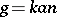of elements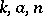of analytic subgroups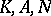, respectively, where,andare defined as follows. Let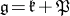be a Cartan decomposition of the Lie algebraof; let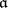be the maximal commutative subspace of the space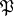, and let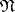be a nilpotent Lie subalgebra ofsuch thatis the linear hull of the root vectors in some system of positive roots with respect to. The decomposition of the Lie algebra as the direct sum of the subalgebras,andis called the Iwasawa decomposition  of the semi-simple real Lie algebra. The groups,andare defined to be the analytic subgroups ofcorresponding to the subalgebras,and, respectively. The groups,andare closed;andare simply-connected;contains the centre of, and the image ofunder the adjoint representation ofis a maximal compact subgroup of the adjoint group of. The mappingis an analytic diffeomorphism of the manifold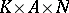onto the Lie group. The Iwasawa decomposition plays a fundamental part in the representation theory of semi-simple Lie groups. The Iwasawa decomposition can be defined also for connected semi-simple algebraic groups over a-adic field (or, more generally, for groups of-adic type) (see , ).